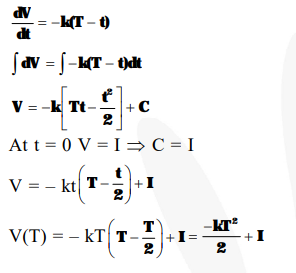# Let I be the purchase value of an equipment and

Question:

Let I be the purchase value of an equipment and $\mathrm{V}(\mathrm{t})$ be the value after it has been used for $t$ years. The value $V(t)$ depreciates at a rate given by differential equation $\frac{\mathrm{dV}(\mathrm{t})}{\mathrm{dt}}=-\mathrm{k}(\mathrm{T}-\mathrm{t})$,

where $\mathrm{k}>0$ is a constant and $\mathrm{T}$ is the total life in years of the equipment. Then the scrap value $V(T)$ of the equipment is :-

1. $I-\frac{k(T-t)^{2}}{2}$

2. $e^{-k T}$

3. $T^{2}-\frac{I}{k}$

4. $\mathrm{I}-\frac{\mathrm{kT}^{2}}{2}$

Correct Option: , 4

Solution: